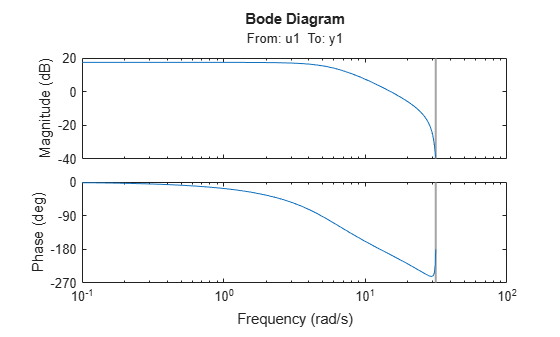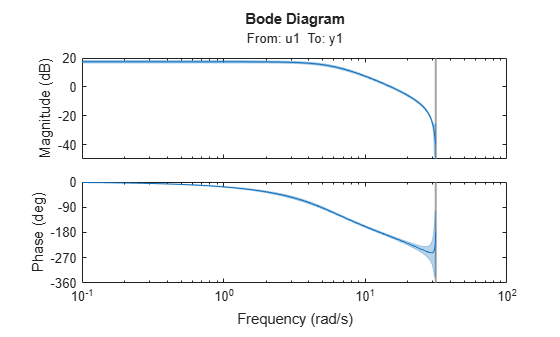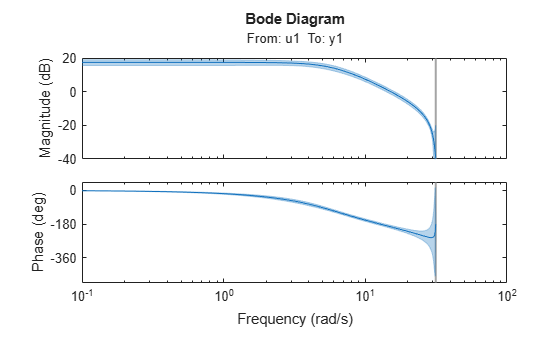# showConfidence

Display confidence regions on response plots for identified models

## Syntax

``showConfidence(plotHandle)``
``showConfidence(plotHandle,stdDev)``

## Description

example

````showConfidence(plotHandle)` displays the confidence region on the response plot for an identified model using a default standard deviation of `1`. ```

example

````showConfidence(plotHandle,stdDev)` displays the confidence region for `sd` standard deviations.```

## Examples

collapse all

Show the confidence bounds on the bode plot of an identified ARX model.

Obtain identified model and plot its bode response.

```load iddata1 z1 sys = arx(z1, [2 2 1]); h = bodeplot(sys);````z1` is an `iddata` object that contains time domain system response data. `sys` is an `idpoly` model containing the identified polynomial model. `h` is the plot handle for the bode response plot of `sys`.

Show the confidence bounds for `sys`.

`showConfidence(h);`This plot depicts the confidence region for 1 standard deviation.

Show the confidence bounds on the bode plot of an identified ARX model.

Obtain identified model and plot its bode response.

```load iddata1 z1 sys = arx(z1, [2 2 1]); h = bodeplot(sys);````z1` is an `iddata` object that contains time domain system response data. `sys` is an `idpoly` model containing the identified polynomial model. `h` is the plot handle for the bode response plot of `sys`.

Show the confidence bounds for `sys` using 2 standard deviations.

```sd = 2; showConfidence(h,sd);````sd` specifies the number of standard deviations for the confidence region displayed on the plot.

## Input Arguments

collapse all

Plot handle for a response plot of an identified model, specified as a `handle` object. To obtain the plot handle, use the `bodeplot`, `stepplot`, `impulseplot`, `nyquistplot`, or `iopzplot` function.

Standard deviation of confidence region, specified as a positive scalar. A common choice is three standard deviations, which gives 99.7% significance.

## Alternatives

You can interactively enable the confidence region display on a response plot. Right-click the response plot, and select Characteristics > Confidence Region.

## Version History

Introduced in R2012a